Homework Help Question & Answers

# Problem 2 (60 POINTS) (20 Points for each question) Fig 1a shows a hollow tube with...

Problem 2 (60 POINTS)
(20 Points for each question)
Fig 1a shows a hollow tube with internal diameter of 230 mm and thickness of 30 mm, as shown in Fig 1b. The length of the tube is 1m.
Fig 1c shows a solid circular bar which also has the same length and the same weight as the hollow circular bar. Both the bars are made of same material (have same density or specific weight)
Fig 1a
Fig 1b Fig 1c
a) Calculate the radius of the solid circular bar.
b) Calculate the Polar moment of Inertia for both the bars.
c) Mention which one has a bigger value of Polar Moment of Inertia (J). Explain in details the significance
of having a bigger value of J considering both the bars are only under torsional load. Your explanation may be supported by formulas and illustrations.

#### Homework Answers

Answer #1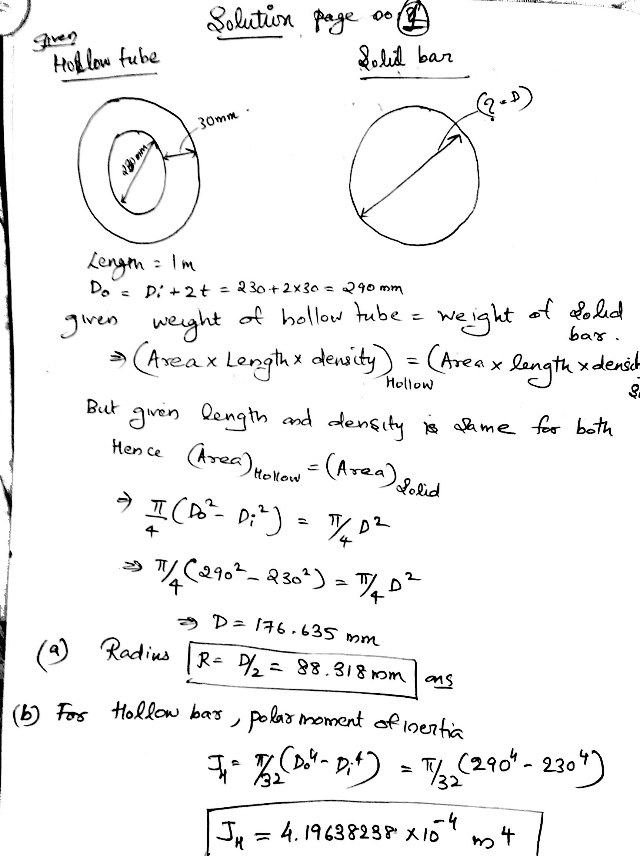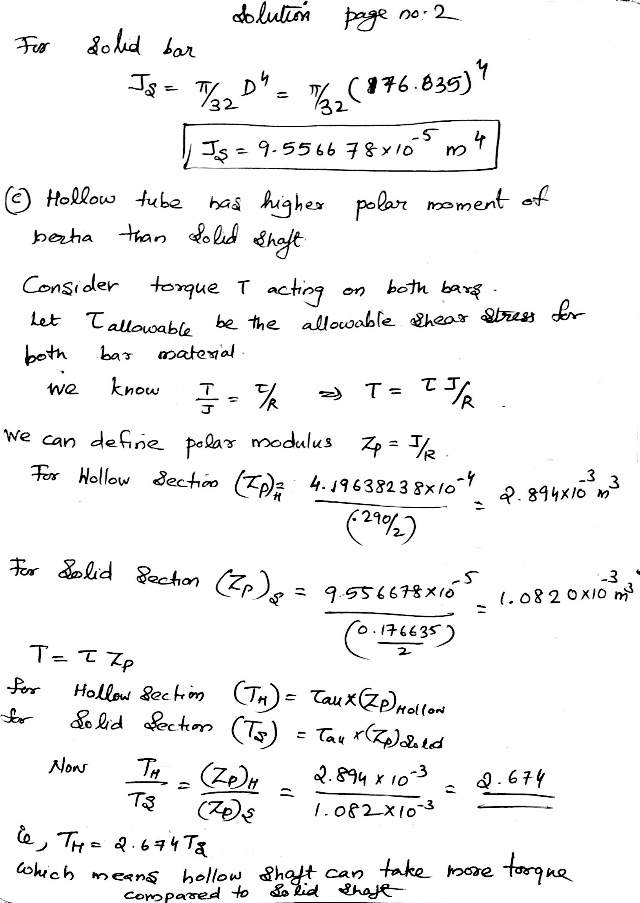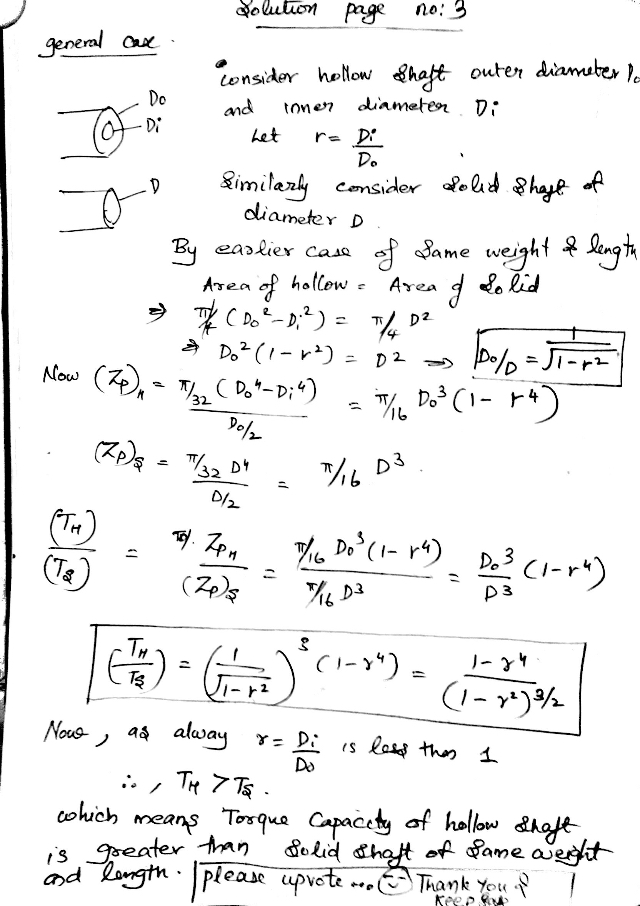Know the answer?
Your Answer:

#### Post as a guest

Your Name:

What's your source?

#### Earn Coin

Coins can be redeemed for fabulous gifts.

Not the answer you're looking for? Ask your own homework help question. Our experts will answer your question WITHIN MINUTES for Free.
Similar Homework Help Questions
• ### 60 min 30 mm 2. Problem #2: (30 points) A torque T = 3kN-m is applied...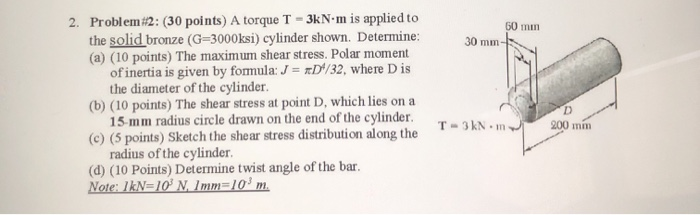60 min 30 mm 2. Problem #2: (30 points) A torque T = 3kN-m is applied to the solid bronze (G=3000ksi) cylinder shown. Determine: (a) (10 points) The maximum shear stress. Polar moment of inertia is given by formula: J = #D/32, where Dis the diameter of the cylinder. (b) (10 points) The shear stress at point D, which lies on a 15-mm radius circle drawn on the end of the cylinder. (c) (5 points) Sketch the shear stress distribution...

• ### Question 1 The motor shown in Fig. 1 supplies power to Gear C through solid steel...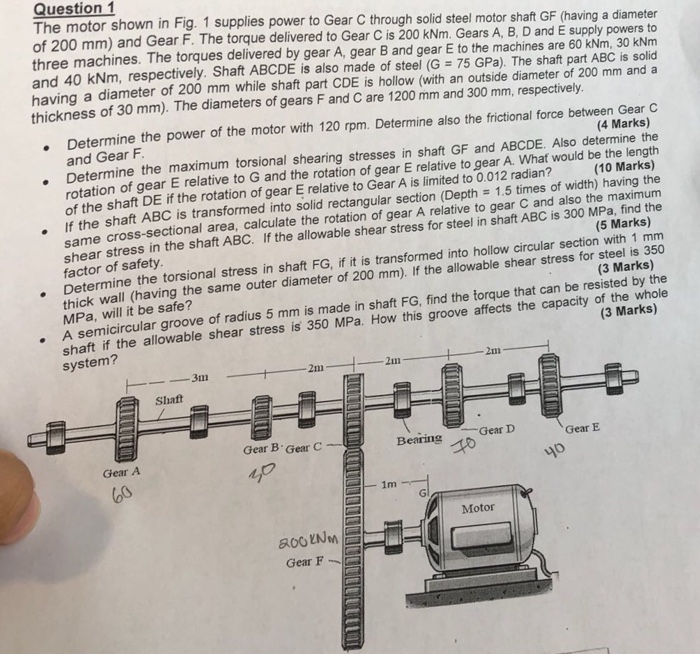Question 1 The motor shown in Fig. 1 supplies power to Gear C through solid steel motor shaft GF (having a diamet of 200 mm) and Gear F. The torque delivered to Gear C is 200 kNm. Gears A, B, D and E supply powers to er three machines. The torques delivered by gear A, gear B and gear E to the machines are 60 kNm, 30 kNm and 40 kNm, respectively. Shaft ABCDE is also made of steel (G...

Free Homework App

Scan Your Homework
to Get Instant Free Answers
Need Online Homework Help?

Get Answers For Free
Most questions answered within 3 hours.